# Binary Trees Interview Questions & Tips

By Kenny Polyak | Published: June 27, 2023

## What is a Binary Tree?

A binary tree is a type of tree data structure where each node can have at most two children, typically referred to as the left child and the right child. This binary structure allows for efficient searching, insertion, and deletion operations, especially when further rules are applied to the tree to express different types of binary trees.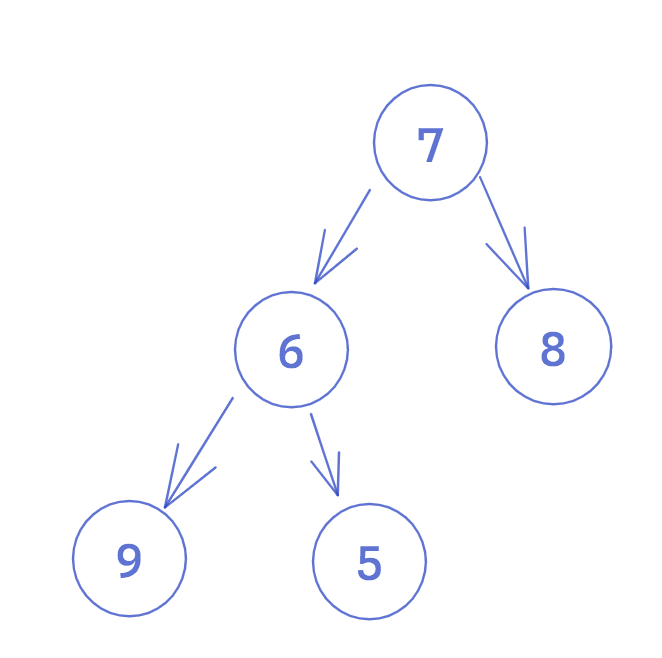Here's an example of a binary tree node implementation:

``````class BinaryTreeNode {
Integer data = null;
BinaryTreeNode left = null;
BinaryTreeNode right = null;

BinaryTreeNode(Integer value) {
data = value;
}
}``````

Given that not all nodes need to have two children, binary trees can be tall or wide, and everything in between. If each node in a binary tree only has one child (except for leaves), the tree would be much taller than it is wide. On the other hand, if most or all the nodes in a binary tree have two children, then the tree would be considered balanced.

Specifically, we can view binary trees as being balanced or unbalanced by this measure: a binary tree is balanced when the heights of the left and right subtrees of any node differ by at most one. **The height of a tree is determined by the number of edges in the longest path from the root to a leaf. **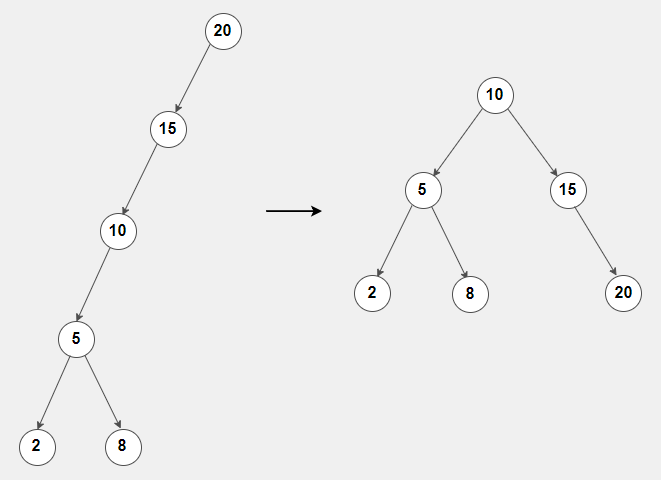The main advantage of a balanced binary tree is that we can achieve optimal performance for searching, adding and deleting operations - by maintaining logarithmic height, these operations can be performed in O(log n) time complexity on average.

Examples of balanced binary trees are AVL trees and Red-Black trees. These are considered advanced topics, sometimes found in database implementations along with other use-cases, and rarely come up in interview questions. More often, when discussing binary tree optimizations, we encounter binary search trees.

## What is a Binary Search Tree (BST)?

A common implementation of a binary tree is a binary search tree. Right there in the name, the binary search tree enables efficient implementation of the binary search algorithm thanks to the way the binary tree is organized: for each node in the tree, the value of the node is greater than the value of all the nodes in its left subtree and smaller than the value of all the nodes in its right subtree.Due to the tree's binary search property, this structure enables a systematic and efficient search process. When searching for a target value, comparisons are made at each node to determine whether to process the left or the right subtree. This allows for the elimination of half of the remaining search space at each step, resulting in a worst-case time complexity of O(log n) for searching, where n is the number of nodes in the tree.

The height of a binary search tree affects the efficiency of the operations. Balanced binary search trees, such as AVL trees or Red-Black trees, maintain a balanced structure to ensure logarithmic time complexity for operations.

### Implementing a Binary Search Tree

To create a binary search tree, the insertNode method will enforce the binary search property. Traversing from the root, insertNode will recursively search for the correct position to add the new node by checking the binary condition: if the currentNode is larger than the new node, traverse left, if the currentNode is smaller than the new node, traverse right. Search will apply the same logic, but return if the target is found.

#### Insert

``````private BinaryTreeNode insertNode(BinaryTreeNode root, int key) {
if (root == null) {
return new BinaryTreeNode(key);
}

if (key < root.data) {
root.left = insertNode(root.left, key);
} else if (key > root.data) {
root.right = insertNode(root.right, key);
}

// if the input key already exists, we don't do anything.
return currentNode;
}``````

#### Delete

``````private BinaryTreeNode deleteNode(BinaryTreeNode root, Integer key) {
if (root == null) {
return root;
}

if (key < root.data) {
root.left = deleteNode(root.left, key);
} else if (data > root.data) {
root.right = deleteNode(root.right, key);
} else {
// Node to be deleted is found

// Case 1: Node has no child or only one child
if (root.left == null) {
return root.right;
} else if (root.right == null) {
return root.left;
}

// Case 2: Node has two children
root.data = minValue(root.right);
root.right = deleteNode(root.right, root.data);
}

return root;
}

private Integer minValue(BinaryTreeNode root) {
Integer minValue = root.data;
while (root.left != null) {
minValue = root.left.data;
root = root.left
}
return minValue;
}``````

## Traversal Order in a Binary Tree

A common task with binary trees is traversing the data structure, since without random access, this is the only way to do anything with our data: search, add, delete, print, etc. In addition to selecting an appropriate traversal algorithm, we also need to determine the order in which we want to visit the nodes.

At a high level, there are two types of traversals: depth-first search (DFS) and breadth-first search (BFS). To explore these algorithms generally, you should read more about DFS and BFS. But in this article, we'll specifically discuss how traversal order is important for binary tree traversal.

DFS is a search algorithm that traverses a tree data structure by prioritizing exploring deeper paths from from child node to child node until a leaf node is finally visited or some condition is met. When visiting each node in a binary tree, the DFS algorithm has three operations it needs to perform in some order: "visit the node", which means perform some work (eg. print the value, add to some counter, delete it, etc), traverse down the left subtree, and traverse down the right subtree. The order of these three operations has a huge impact on the ultimate traversal order, so we further subdivide DFS into preorder, inorder, and postorder traversal.

As an alternative to DFS, the BFS algorithm prioritizes visiting all the direct children at the same level before moving deeper into the tree. With this pattern, there is only one possible traversal order, which is called level-order traversal.

Let's explore these traversal orders more closely.

### Depth-First Search (DFS)Consider an example where we are printing all the nodes of the binary search tree from before using a DFS traversal.

#### Inorder Traversal

Inorder traversal is a process for visiting each node in a binary tree by first visiting the left subtree, then the node itself, and then the right subtree. With inorder traversal, the path always favors the leftmost tree before traversing the rest.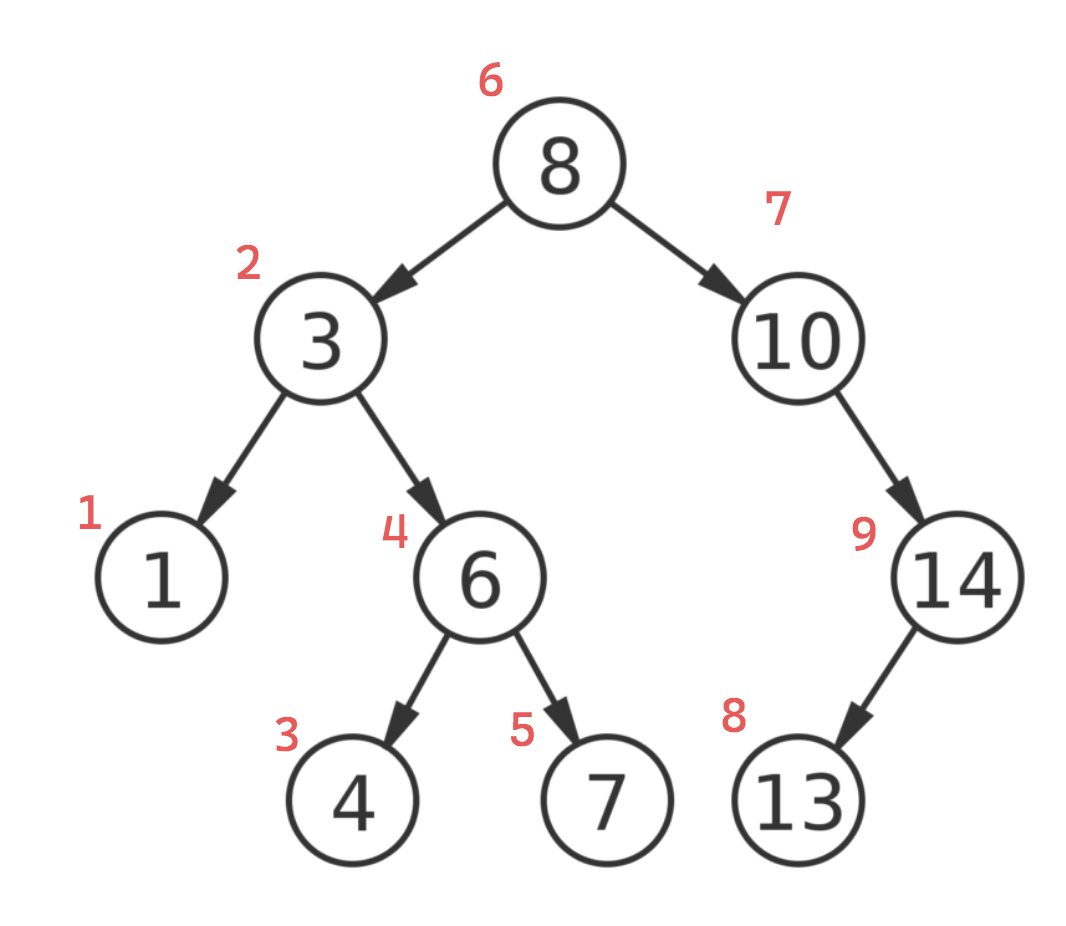The sequence produced with inorder traversal: 1, 3, 4, 6, 7, 8, 10, 13, 14.

In a binary search tree, inorder traversal results in visiting the nodes in ascending order. This is because by favoring resolving the left subtree at each node, at each node we are always moving toward the smallest value available and returning the inorder successor.

``````void inorderTraversal(BinaryTreeNode node) {
// base case: if node is null, do nothing
if (node != null) {
// recurse on left child
inorderTraversal(node.left);

// visit current node
System.out.print(node.data + " ");

// recurse on right child
inorderTraversal(node.right);
}
}``````

#### Preorder Traversal

Preorder traversal visits each node in the tree by first visiting the node itself, then traversing the left subtree, and finally traversing the right subtree. In each recursive call, the function first prints (or "visits") the current node, then calls the recursive function on the left subtree, and finally on the right subtree.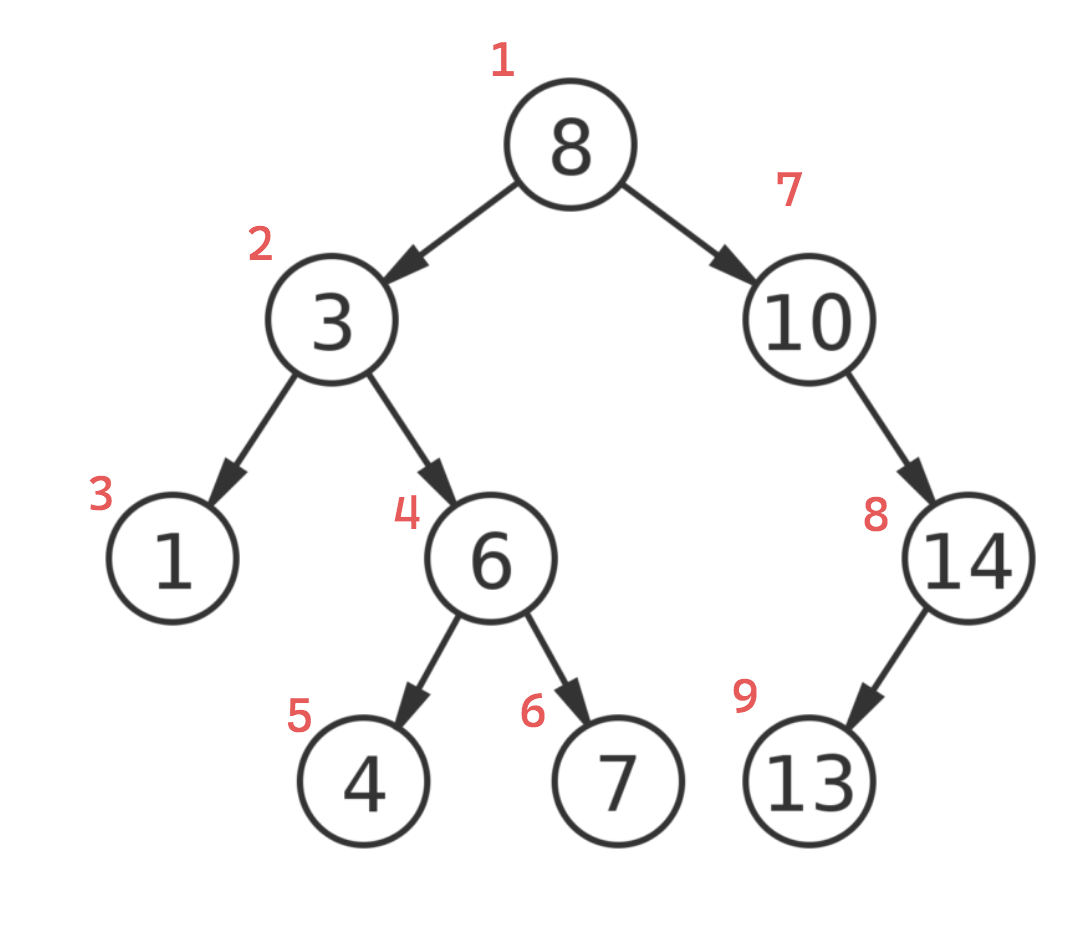The sequence produced with preorder traversal: 8, 3, 1, 6, 4, 7, 10, 14, 13

``````void preorderTraversal(BinaryTreeNode node) {
// base case: if node is null, do nothing
if (node != null) {
// visit current node
System.out.print(node.data + " ");

// recurse on left child
preorderTraversal(node.left);

// recurse on right child
preorderTraversal(node.right);
}
}``````

#### Postorder Traversal

In each recursive call, the function first performs DFS on the left subtree, then performs DFS on the right subtree, and finally visits the current node.The sequence produced with postorder traversal: 7, 6, 4, 1, 3, 13, 14, 8

``````void postorderTraversal(BinaryTreeNode node) {
// base case: if node is null, do nothing
if (node != null) {
// recurse on left child
postorderTraversal(node.left);

// recurse on right child
postorderTraversal(node.right);

// visit current node
System.out.print(node.data + " ");
}
}``````

Postorder traversal is often used to delete the nodes of a tree in a specific order, because we can easily reconstruct the node references. We are basically marking the exact path of the recursive calls by immediately printing each node as it is visited.

#### Level Order Traversal

As an alternative to using DFS we can also traverse a binary tree using Breadth-First Search (BFS), where we visit each node belonging to the same level before moving deeper into the tree. BFS uses a queue data structure (instead of a stack or recursion), in order to maintain the level-order traversal.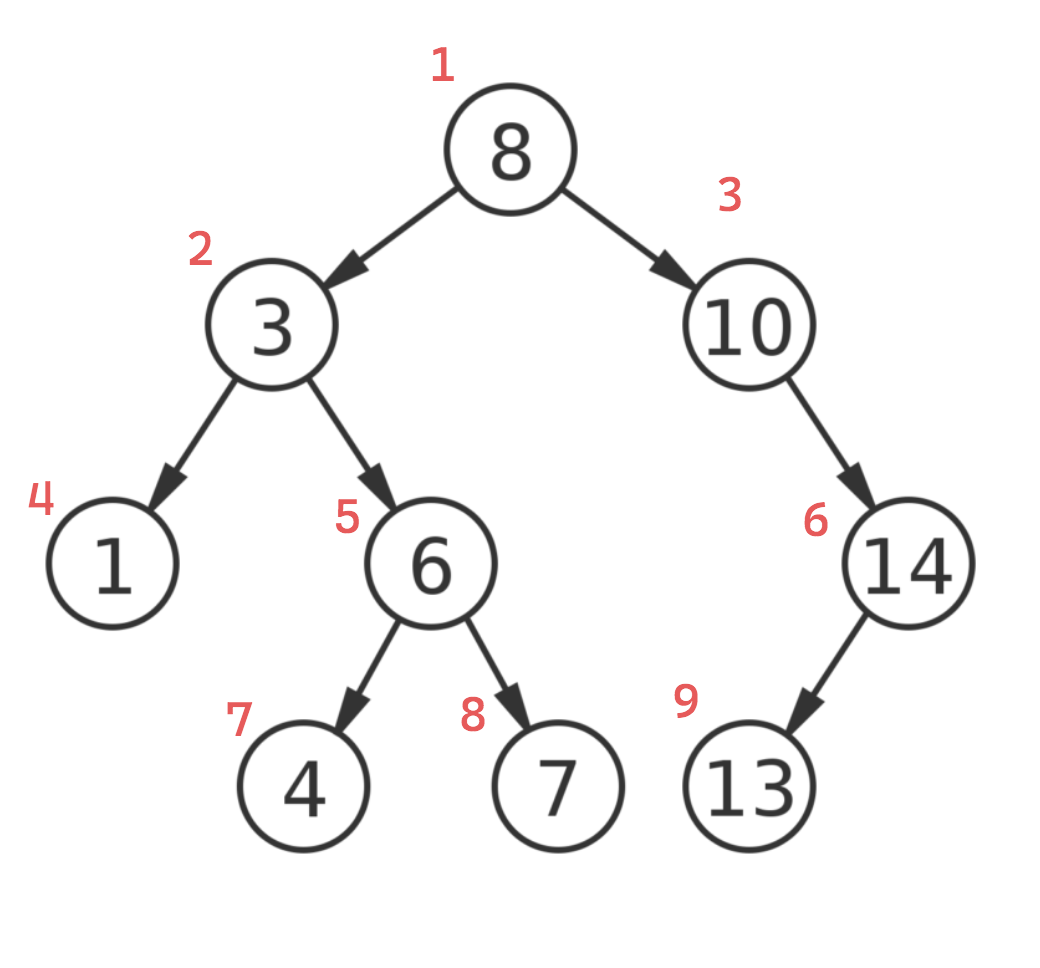The sequence produced with level order traversal: 8, 3, 10, 1, 6, 14, 4, 7, 13

Level order traversal in a binary tree is often applied to problems where we need to process tree nodes by level, or if we want to find the shortest distance between two nodes.

``````public static void levelOrderTraversal(TreeNode root) {
if (root == null)
return;

Queue < TreeNode > queue = new LinkedList < > ();
queue.offer(root);

while (!queue.isEmpty()) {
TreeNode node = queue.poll();
System.out.print(node.val + " ");

if (node.left != null)
queue.offer(node.left);

if (node.right != null)
queue.offer(node.right);
}
}``````

### Time and Space Complexity

Time complexity: `O(n)`, where n is the number of nodes. If we're not explicitly performing binary search, we will visit every node at worst in a traversal.

Space complexity: `O(n)`, additional space is needed on the call stack when performing recursion.

## When to Use Binary Trees In Technical Interviews

Most of the time, interview questions involving trees will be explicitly stated as such. The problem will come in the form of “Given a tree, do X”. Sometimes, the task may be challenging but not very ambiguous, for example validating a binary search tree. The most important thing when you see problems like this is to make sure that you understand what type of tree you’re dealing with. If it’s a BST, that has different implications than a binary tree that is not sorted, and could provide valuable clues for arriving at an optimal solution.

In other cases, we might be asked to store data efficiently - this could be an opportunity to implement a BST. A common interview task is to implement the insertion and search functions of a BST, as this is a great way to demonstrate one's understanding of the data structure, so be sure to practice these. Deleting a node from a BST can be asked as well but is often considered an advanced topic.

For generic binary trees, questions often involve assessing the dimensions of the tree, for example the height or diameter of the tree, or searching specific conditions between two or more nodes in the tree, like LCA or path sum. Here are some areas that come up often in interviews:

1. Height: Calculate the height of a binary tree (the number of edges on the longest path from the root to a leaf node).
2. Find Mirror Image: Determine if a binary tree is a mirror image of itself (symmetric).
3. Lowest Common Ancestor (LCA): Given two nodes in a binary tree, find their lowest common ancestor node.
4. Diameter of a Tree: Calculate the diameter of a binary tree (the length of the longest path between any two nodes).
5. Path Sum: Check if there exists a root-to-leaf path in a binary tree that adds up to a given sum. 6.Serialize and Deserialize: Serialize a binary tree into a string representation and deserialize it back to a binary tree.

## Common Mistakes in Interviews Featuring Binary Trees

• Mistaking a Binary Tree for a Binary Search Tree. Remember that an interviewer might intentionally leave information out of their problem description to leave room for your inquiries. The difference between a general binary tree and a BST will greatly influence the solution you propose.
• Forgetting to consider duplicate keys when implementing a BST. Paying close attention to implementation details will help demonstrate your familiarity with the data structure.
• Not using visual aids. Binary tree logic can become very complex, given its recursive nature. Using tree diagrams can help you work through the problem and communicate more effectively with your interviewer.
• Misusing BFS or DFS. In some binary tree problems, where we don't have a sorted tree or we simply need to visit every node to perform some operation, both traversal algorithms are applicable without any meaningful complexity tradeoff. But a candidate needs to be confident about which situations call for a specific traversal. A common use-case for BFS, for example, is searching for the shortest path between two nodes. DFS on the other hand, is useful to perform on a binary search tree when we want to traverse the nodes in their sorted order.
• Forgetting to set min/max bounds when validating binary search trees. An incorrect implementation just checks whether node.right.data > node.left.data and node.left.data < node.right.data
• Not knowing how to use recursion within trees. Trees are inherently recursive data structures, so it's important to be familiar with recursive traversal. It may be obvious how to traverse from parent to child, but using recursive to traverse from child to parent (with return statement) is essential.
• Incorrectly stating space complexity for recursive traversal. It is easy to forget that recursion doesn't use additional space since we are not introducing a new data structure. But in fact, we are taking advantage of an existing stack call the call stack, which must grow linearly with the number of nodes we are recursing on.
• Forgetting to handle edge cases. Binary tree nodes can still have zero or one child, so be sure to explicitly check for edge cases. We also need to include base cases for recursive traversals.

• Is the input binary tree or a binary search tree? Clarifying the parameters offered by the interviewer is a great problem-solving skill to demonstrate. In some cases, interviewers will intentionally omit that the binary tree you're working with is actually sorted, so be sure to ask! This of course will have a huge impact on the approach you'll end up taking for your solution.
• Will the input contain duplicate values? Whether you are streaming values or getting a tree as input, make sure to specify if duplicates need to be handled, as this complicates binary search tree implementation. For binary search trees, this is especially complicated, and will likely be the crux of the problem if the tree contains them. Alternatively, you might be building a BST from a stream of values, and you'll want to be sure you can omit duplicates if its appropriate in the problem.
• How do we handle scenarios where a binary tree is empty or has null nodes? It is always encouraged to ask about how edge cases should be handled, as some interviewers will be happy enough that you communicated that you are aware of them, and will offer to let you skip implementation.
• What operations need to be supported? If you'll be implementing a binary tree, make sure to ask your interviewer what operations to prioritize during the interview. In some cases, they can allow you to skip the implementation of some less-important operations.
• What are the characteristics of the input tree? Be sure to determine if there are any constraints that the input binary tree adheres to, such as balancing or sorting, max height, weighted branches, etc. If so, this would be a clue as to what kind of tree data structure you should focus on during the interview.

## How to Show Mastery of Trees in Interviews

One of the most common topics in software engineering interviews is the Binary Search Tree. You want to be able to showcase your ability to implement binary trees efficiently and correctly. Make sure to practice implementing tree construction, node insertion, deletion, and traversal algorithms.

Speaking of traversal algorithms - many interview problems test your understanding of the traversal order, especially when working with binary search trees, since the output sequence order is not arbitrary. Be sure to understand the use-cases for preorder, inorder, postorder, and level-order traversals.

### Be Familiar with Recursive and Iterative Implementations of DFS

Although trees are inherently recursive, and thus lend themselves to recursive traversal implementations, a candidate should be comfortable with the iterative implementation as well. This helps demonstrate your strong understanding of recursion as well, since we can mimic the recursive mechanism we get from the call stack with a stack we implement ourselves. The above traversals are all recursive - here's an example of an iterative DFS:

#### Iterative DFS

``````public static void iterativeDFS(BinaryTreeNode root) {
if (root == null)
return;

Stack < BinaryTreeNode > stack = new Stack < > ();
stack.push(root);

while (!stack.isEmpty()) {
BinaryTreeNode node = stack.pop();
System.out.print(node.val + " ");

// Push right child first (since it needs to be processed after left child)
if (node.right != null)
stack.push(node.right);

// Push left child
if (node.left != null)
stack.push(node.left);
}
}``````

## Adjacent Topics to Binary TreesKenny Polyak

Kenny is a software engineer and technical leader with four years of professional experience spanning Amazon, Wayfair, and U.S. Digital Response. He has taught courses on Data Structures and Algorithms at Galvanize, helping over 30 students land new software engineering roles across the industry, and has personally received offers from Google, Square, and TikTok.